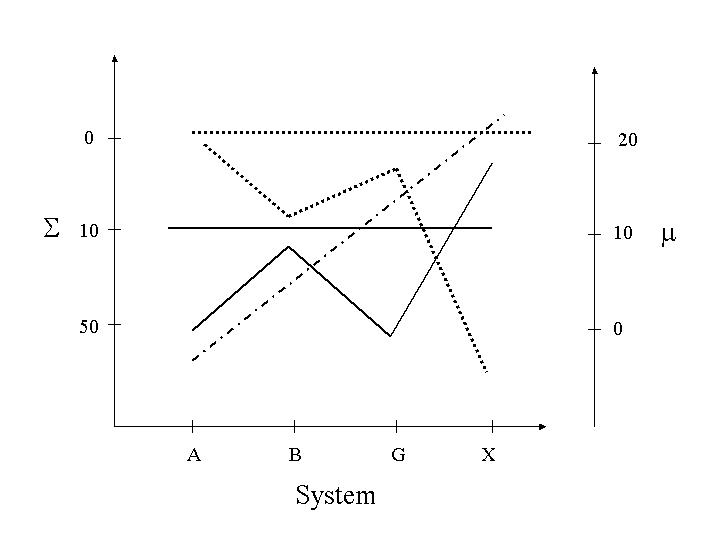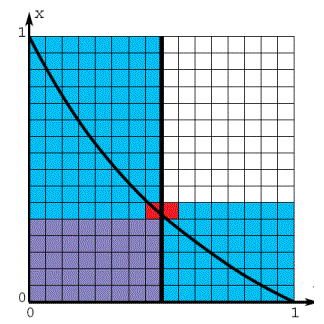# CS533 Sample Mid-Term Exam Questions

1. Briefly describe the three broad categories of performance evaluation techniques. Provide a positive and a negative characteristic for each.

2.Fill in the ??? in the above table.

3. Given the following response times from a database transaction server (in milliseconds): 0.21, 0.55, 0.20, 1.25, 162.18, 7860, 7721, 20740, 22360, 24440, 28560

1. What index of central tendency would you use to describe the data? Briefly explain why.
2. What index of dispersion would you use to describe the data? Briefly explain why.

4. Given the performance of system A and system B under two different workloads:

```  System    Workload 1    Workload 2
----------------------------------
A         10            30
B         20            20
```
Use a ratio game to show that system B is better.

5. What is the main hypothesis in LHPT95? Briefly describe the methodology they use to test their hypothesis.

6. What is at least one problem with instruction mixes as a computer workload? What is at least one problem with a kernel as a computer workload? What is at least one problem with an application as a computer workload?

7. What are the primary differences between hardware and software monitors?

8. The CCC is trying to characterize the workload on their across-campus Web machines. Several student projects have produced the following workload components (CPU in ms, disk in requests):

```  Program Name    Function         CPU  Disk
Emacs           Editor           10   0
Pine            Email            20   100
Netscape        Browser          10   200
Savage          Game             70   200
Gcc             Compiler         50   500
Gnuplot         Graphing         30   100
Povray          Rendering        90   100
```
1. What is the first step you would take in clustering the data for building a workload? Do it.

2. What is the second step you would take in clustering the data? Do it, justifying your answer.

9. Of the audio degradations induced in WS00, why are some of them deemed equivalent?

10.Give at least 5 problems with the above graph.

11.Describe the HINT algorithm (from GS95). Refer to the above graph in your answer.Return to the CS533 Home Page